# Cochrane-Orcutt estimation in Excel tutorial

2017-10-20

This tutorial will help you set up and interpret a Cochrane-Orcutt model on a linear model with autocorrelated residuals in Excel using XLSTAT.

## Dataset to run a Cochrane-Orcutt model in Excel

The data correspond to the consumer expenditure and money stock example of  Chatterjee et al. [Chatterjee, S., Hadi, A.S. and Price, B. (2000). Regression Analysis by Example, Wiley-Interscience Publication,John Wiley and Sons]. In this example, Household consumption is the dependent variable and money stock the explanatory one.

## Goal of this tutorial

Assume that the error term of the linear model is serially correlated, we want to use the Cochrane-Orcutt estimation.

## Setting up the Cochrane-Orcutt estimation

After opening XLSTAT, select the XLSTAT / Time / Cochrane-Orcutt command.

Once you've clicked on the button, the dialog box appears.

The data are presented in a table of 20 observations and 2 variables. The Expend is the dependent variable and the Stock is the explanatory variable. As we selected the column title for the variables, we leave the option Variable labels activated.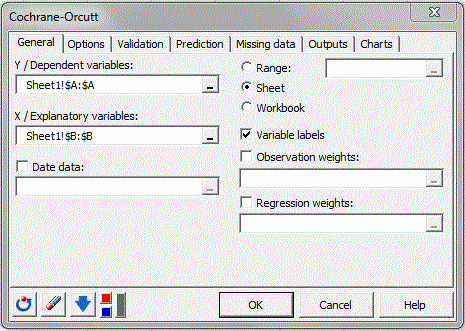On the Options tab, the user can set the tolerance and the level of confidence intervals. Here we choose to leave the default values.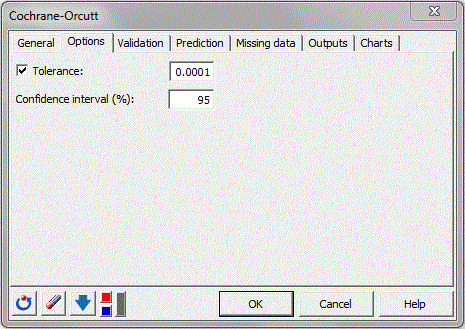The computations begin once you have clicked OK. The results will then be displayed in a new sheet.

## Interpreting the results

The first results displayed are the statistics for the various variables. Next, a table displays the goodness of fit coefficients of the model. The R² (coefficient of determination) indicates the % of variability of the dependent variable which is explained by the explanatory variables. The closer to 1 the R² is, the better the fit.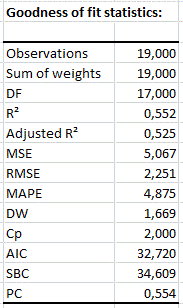It is important to examine the results of the analysis of variance table (see below). The results enable us to determine whether or not the explanatory variable bring significant information (null hypothesis H0) to the model. In other words, it's a way of asking yourself whether it is correct to use the mean to describe the whole population, or whether the information brought by the explanatory variable is of value or not.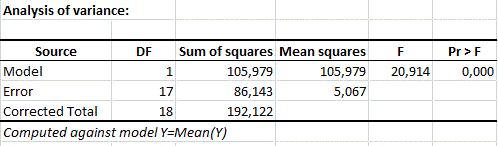The following tables give details on the model (parameters, sd, …) and the autocorrelation coefficient rho. These tables are helpful when predictions are needed, or when you need to compare the coefficients of the model for a given population with the ones obtained for another population.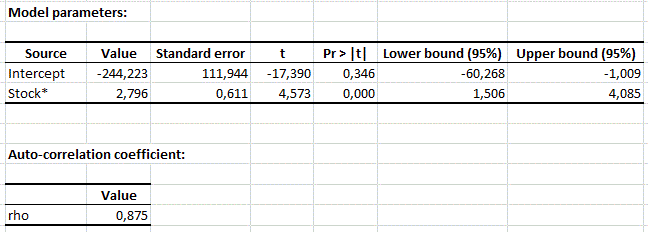Next, tables and graphs enable us to take a closer look at each of the standardized residuals. These residuals, should be normally distributed, meaning that 95% of the residuals should be in the interval [-1.96, 1.96]. The histogram of the residuals enables us to quickly visualize the residuals that are out of this interval.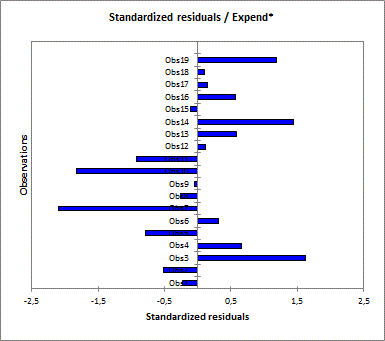Here, only one of the values is out of the interval.

#### Contact our technical support team: support@xlstat.com

https://cdn.desk.com/
false
desk